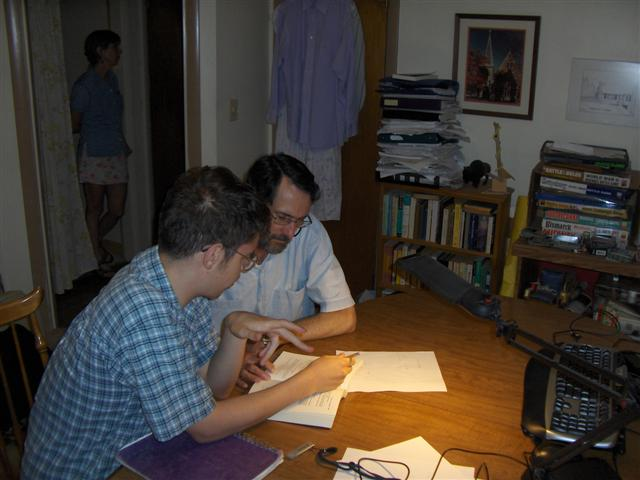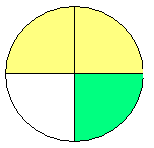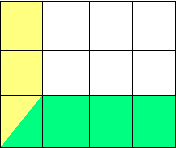## My Approach to Tutoring

To give you an idea of how I work with students, here's an example of a problem that I see very frequently. My student Hannah and I are working on adding fractions. Hannah reads this problem out of her book:

4/5 + 1/7 = ?

Her solution, a very common misconception, is:

4/5 + 1/7 = (4+1)/(5+7) = 5/12

But with a questioning look, she indicates that she knows she's a little hazy on the correct method.

At this point, many people will say "No, that isn't right, to add fractions you have to get a common denominator and add the numerators only." My approach is different. By this time in Hannah's academic career, she's been told dozens of times how to add fractions. The fact that she or her parents have called me in to help tells me that she doesn't understand why, or perhaps what. If you don't understand, you have to fall back on rote memory. And with this example we can see just how lacking rote memory can be.

I know something else too: Hannah either doesn't understand fractions at all, or isn't using her understanding here. After all, 4/5 is nearly 1, and she's adding 1/7, which should make it closer to 1. But her answer, 5/12, is less than 1/2 (which would be 6/12, of course). This answer doesn't pass what I call "the sanity test".

So my first goal is to escape from rote memory and establish the idea that we can use some common sense in arithmetic. I point out that 4/5 is close to 1, that we're adding something to it, and that the answer we have so far is less than what we started with. If I don't get any understanding, then I know that the problem isn't that we don't know how to add fractions; it's that we don't understand the idea of a fraction. In that case, we can back up and work on the more fundamental understanding that's missing.

Once I know that fractions are understood, I can go to the next step of how to add them. I start with something like 1/3 plus 1/3. Most people will pop out with 2/3, duh; but a surprising number need to be guided to the idea that if you have one of something and add it to another of the same thing, you have two of those things. In this case, the "things" we have are thirds, so adding one third to one third gives you two thirds. And now we can see the inconsistency with our earlier addition technique: We don't add tops and bottoms to get

1/3 + 1/3 = (1+1)/(3+3) = 2/6That serves to introduce the idea of obtaining a common denominator where there isn't already one, as in the previous problem. We might do a few more simple problems, depending on the student, but eventually I'll pose something like 1/3 + 1/4. For this I introduce my pan of cornbread (an idea I stole from Dr. Herbert Gross).
 That pan can be divided into thirds along one side, and into fourths along the other, and the common denominator of 12 has appeared as if by magic! Now it's easy for Hannah to see that a third of the pan is 4 of the 12 pieces, and a fourth is three of the 12, so together they're 7 of the 12. Or, if you prefer equations, 1/4 + 1/3 = 3/12 + 4/12 = (3+4)/12 = 7/12